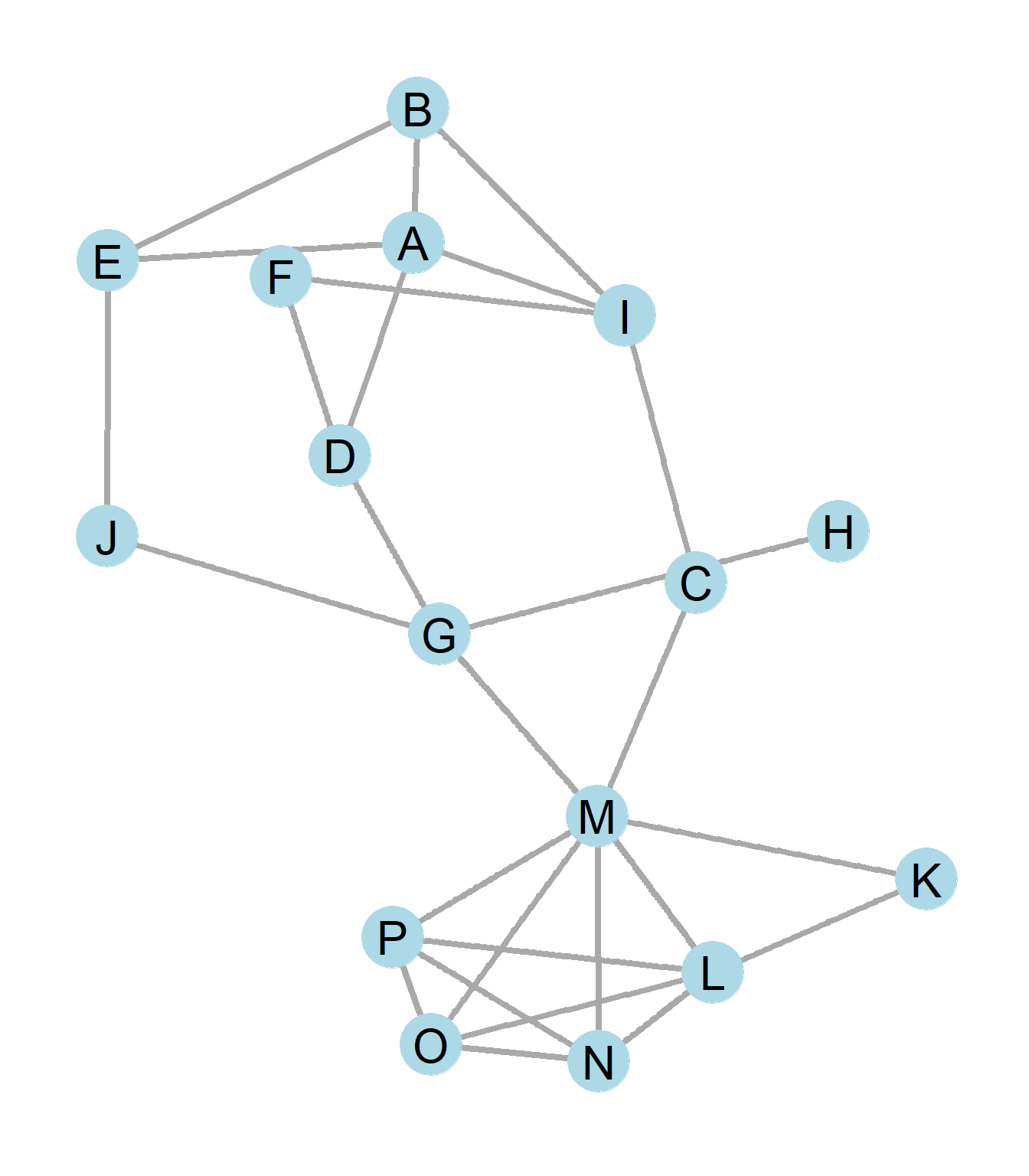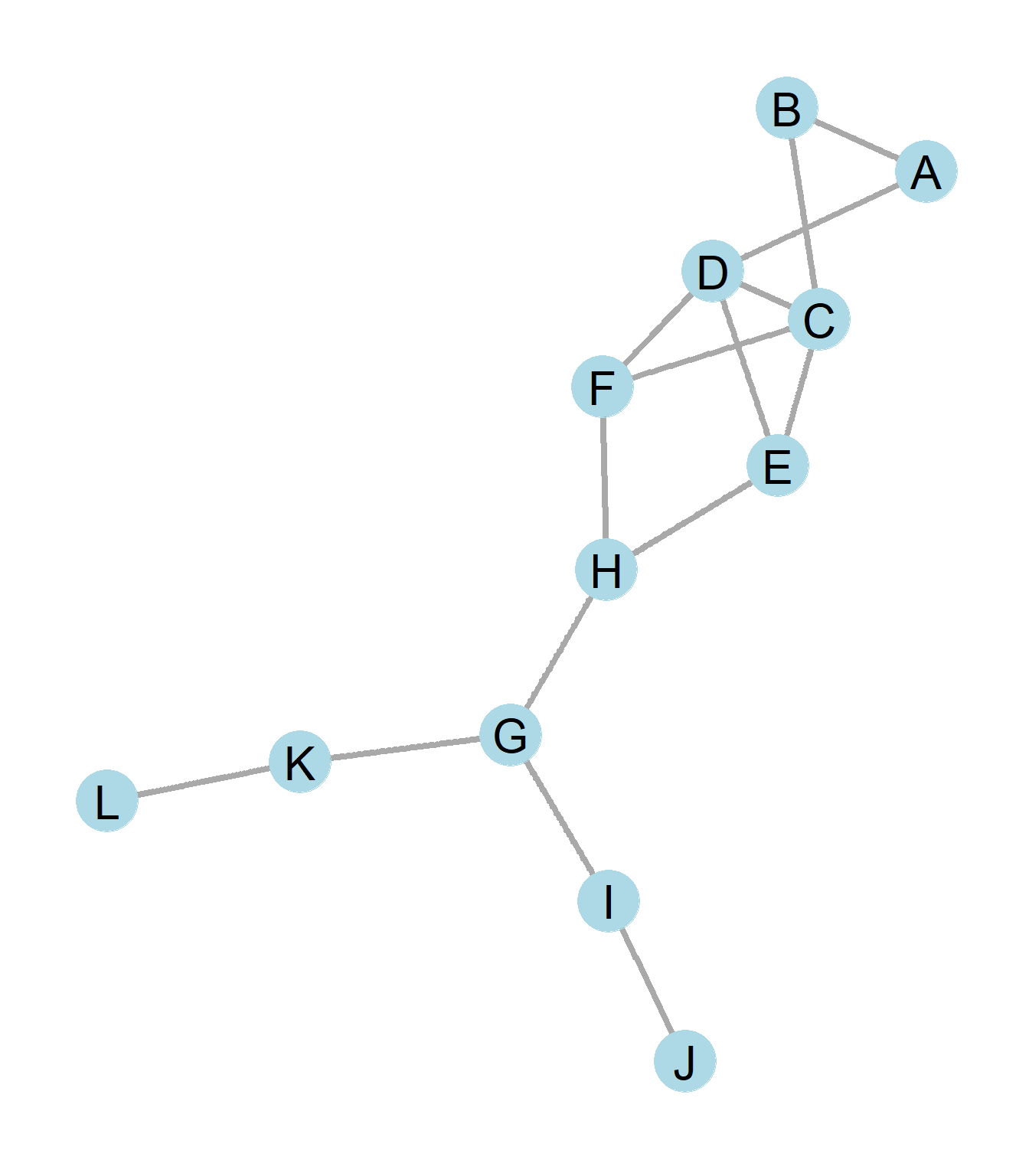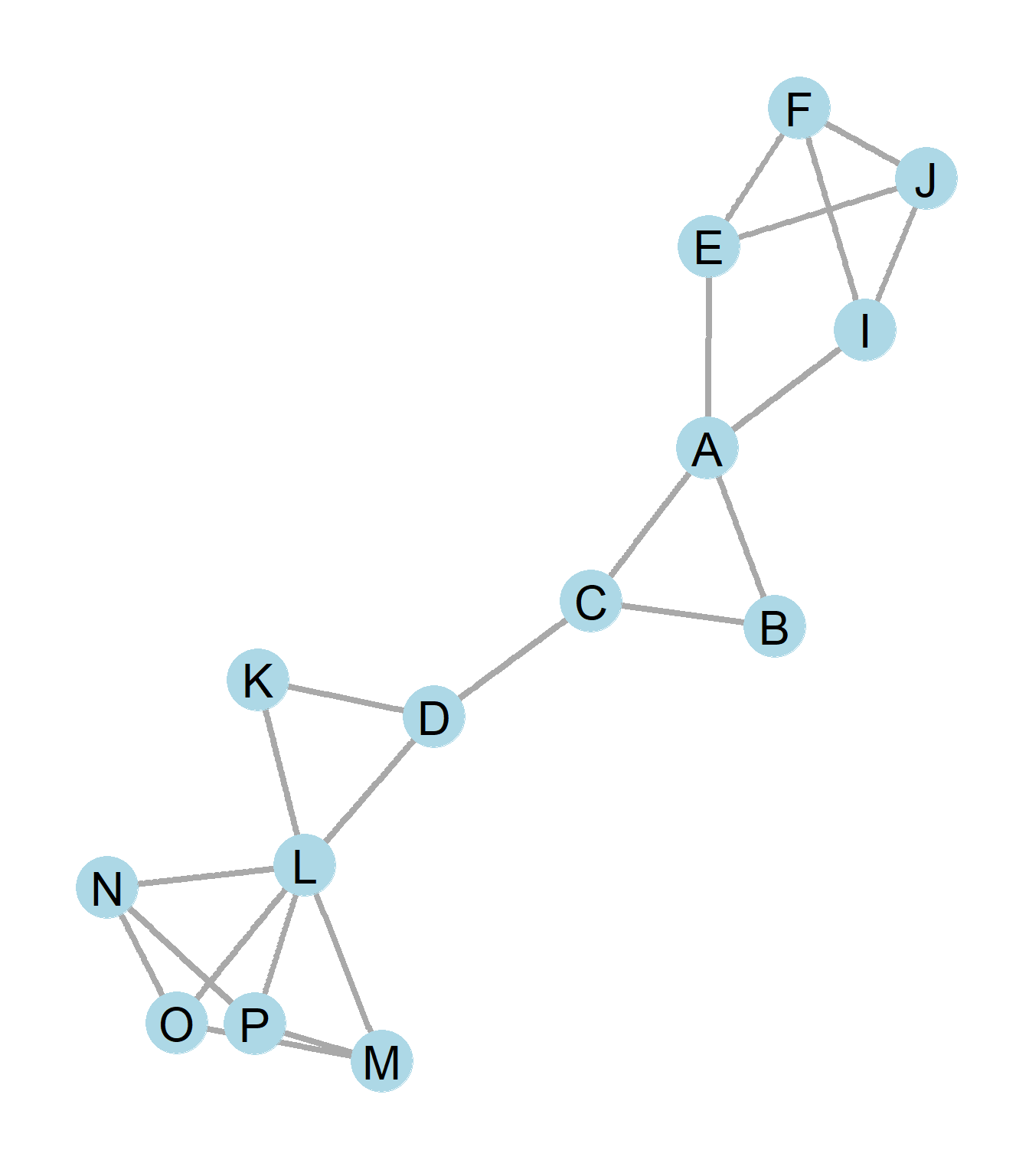## 5.4 Practice Problems

### 5.4.11. The graph is k-connected with what k?
2. What is the diameter of the graph?
3. What is a name for node H? What does it do?
4. Let us remove node H. Notice that we have essentially done some c by removing the fewest node/edges to create network components. What are the resulting n-cliques for each of the resulting components?
5. Calculate the Connectedness score for each of the two components. Which is the more centralized component?

### 5.4.2The Ministry of Silly Walks: Both J and L would like to get approval for funding the development of their new idea, which can only be approved by person A. The bureaucracy to A though is a little convoluted. What is the shortest path for person J and L to get their idea passed up the chain of command for approval?

### 5.4.31. How many components are there in the graph?
2. The giant component is k-connected with what k?
3. What is the diameter of the graph?

Remove component G-H so now the graph is what was before the giant component and answer the following questions. d) What is the diameter of the graph? e) The graph is k-connected with what k? f) What type of clique is subgraph (L, M, N, O, P)? g) What type of clique is subgraph (A, E, I, F, J)?